### Home > CALC > Chapter 6 > Lesson 6.1.1 > Problem6-12

6-12.
1. Examine the integrals below. Consider the multiple tools available for evaluating integrals and use the best strategy for each. After evaluating the integral, write a short description of your method. Homework Help ✎

1.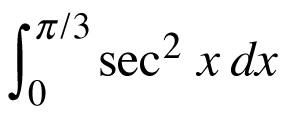2.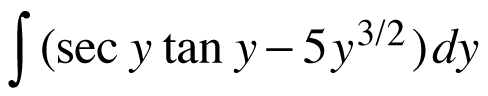3.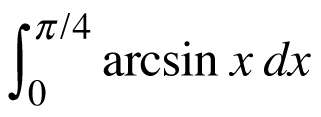4.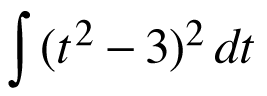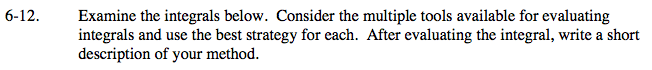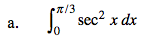$\text{Recall that }\frac{d}{dx}\text{tan}(x)=\text{sec}^{2}(x)$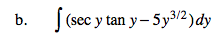$\text{Recall that }\frac{d}{dx}\text{sec}(y)=\text{tan}(y)\text{sec}(y)$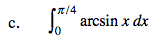≈ 0.328
(By calculator, unless you know the antiderivative
of y = arcsin(x).)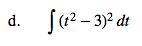Before you integrate, expand the integrand.